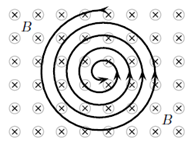# Problem: A static uniform magnetic field is directed into the page. A charged particle moves in the plane of the page following a counterclockwise spiral of decreasing radius as shown. Neglect the effect due to gravity. What is a reasonable explanation? 1. The charge is neutral and speeding up. 2. The charge is positive and with a constant speed. 3. None of these 4. The charge is neutral and with a constant speed. 5. The charge is negative and with a constant speed. 6. The charge is positive and slowing down. 7. The charge is negative and slowing down. 8. The charge is neutral and slowing down. 9. The charge is positive and speeding up. 10. The charge is negative and speeding up.

###### FREE Expert Solution
93% (485 ratings)
###### Problem Details

A static uniform magnetic field is directed into the page. A charged particle moves in the plane of the page following a counterclockwise spiral of decreasing radius as shown. Neglect the effect due to gravity. What is a reasonable explanation?

1. The charge is neutral and speeding up.

2. The charge is positive and with a constant speed.

3. None of these

4. The charge is neutral and with a constant speed.

5. The charge is negative and with a constant speed.

6. The charge is positive and slowing down.

7. The charge is negative and slowing down.

8. The charge is neutral and slowing down.

9. The charge is positive and speeding up.

10. The charge is negative and speeding up.